Newton’s Laws of Motion

# Newton’s Second Law

### Learning Objectives

By the end of the section, you will be able to:

• Distinguish between external and internal forces
• Describe Newton’s second law of motion
• Explain the dependence of acceleration on net force and mass

Newton’s second law is closely related to his first law. It mathematically gives the cause-and-effect relationship between force and changes in motion. Newton’s second law is quantitative and is used extensively to calculate what happens in situations involving a force. Before we can write down Newton’s second law as a simple equation that gives the exact relationship of force, mass, and acceleration, we need to sharpen some ideas we mentioned earlier.

### Force and Acceleration

First, what do we mean by a change in motion? The answer is that a change in motion is equivalent to a change in velocity. A change in velocity means, by definition, that there is acceleration. Newton’s first law says that a net external force causes a change in motion; thus, we see that a net external force causes nonzero acceleration.

We defined external force in Forces as force acting on an object or system that originates outside of the object or system. Let’s consider this concept further. An intuitive notion of external is correct—it is outside the system of interest. For example, in (Figure)(a), the system of interest is the car plus the person within it. The two forces exerted by the two students are external forces. In contrast, an internal force acts between elements of the system. Thus, the force the person in the car exerts to hang on to the steering wheel is an internal force between elements of the system of interest. Only external forces affect the motion of a system, according to Newton’s first law. (The internal forces cancel each other out, as explained in the next section.) Therefore, we must define the boundaries of the system before we can determine which forces are external. Sometimes, the system is obvious, whereas at other times, identifying the boundaries of a system is more subtle. The concept of a system is fundamental to many areas of physics, as is the correct application of Newton’s laws. This concept is revisited many times in the study of physics.

Different forces exerted on the same mass produce different accelerations. (a) Two students push a stalled car. All external forces acting on the car are shown. (b) The forces acting on the car are transferred to a coordinate plane (free-body diagram) for simpler analysis. (c) The tow truck can produce greater external force on the same mass, and thus greater acceleration.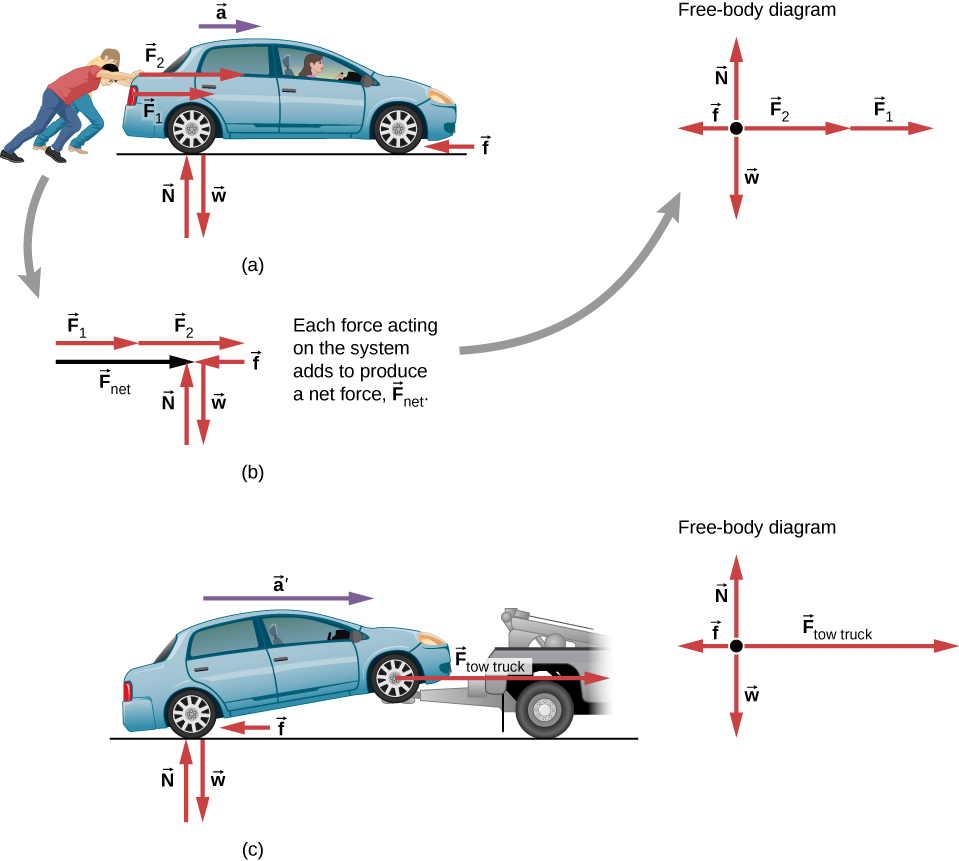From this example, you can see that different forces exerted on the same mass produce different accelerations. In (Figure)(a), the two students push a car with a driver in it. Arrows representing all external forces are shown. The system of interest is the car and its driver. The weight $\stackrel{\to }{w}$ of the system and the support of the ground $\stackrel{\to }{N}$ are also shown for completeness and are assumed to cancel (because there was no vertical motion and no imbalance of forces in the vertical direction to create a change in motion). The vector $\stackrel{\to }{f}$ represents the friction acting on the car, and it acts to the left, opposing the motion of the car. (We discuss friction in more detail in the next chapter.) In (Figure)(b), all external forces acting on the system add together to produce the net force ${\stackrel{\to }{F}}_{\text{net}}.$ The free-body diagram shows all of the forces acting on the system of interest. The dot represents the center of mass of the system. Each force vector extends from this dot. Because there are two forces acting to the right, the vectors are shown collinearly. Finally, in (Figure)(c), a larger net external force produces a larger acceleration $\left(\stackrel{\to }{{a}^{\prime }}>\stackrel{\to }{a}\right)$ when the tow truck pulls the car.

It seems reasonable that acceleration would be directly proportional to and in the same direction as the net external force acting on a system. This assumption has been verified experimentally and is illustrated in (Figure). To obtain an equation for Newton’s second law, we first write the relationship of acceleration $\stackrel{\to }{a}$ and net external force ${\stackrel{\to }{F}}_{\text{net}}$ as the proportionality

$\stackrel{\to }{a}\propto {\stackrel{\to }{F}}_{\text{net}}$

where the symbol $\propto$ means “proportional to.” (Recall from Forces that the net external force is the vector sum of all external forces and is sometimes indicated as $\sum \stackrel{\to }{F}.$) This proportionality shows what we have said in words—acceleration is directly proportional to net external force. Once the system of interest is chosen, identify the external forces and ignore the internal ones. It is a tremendous simplification to disregard the numerous internal forces acting between objects within the system, such as muscular forces within the students’ bodies, let alone the myriad forces between the atoms in the objects. Still, this simplification helps us solve some complex problems.

It also seems reasonable that acceleration should be inversely proportional to the mass of the system. In other words, the larger the mass (the inertia), the smaller the acceleration produced by a given force. As illustrated in (Figure), the same net external force applied to a basketball produces a much smaller acceleration when it is applied to an SUV. The proportionality is written as

$a\propto \frac{1}{m},$

where m is the mass of the system and a is the magnitude of the acceleration. Experiments have shown that acceleration is exactly inversely proportional to mass, just as it is directly proportional to net external force.

The same force exerted on systems of different masses produces different accelerations. (a) A basketball player pushes on a basketball to make a pass. (Ignore the effect of gravity on the ball.) (b) The same player exerts an identical force on a stalled SUV and produces far less acceleration. (c) The free-body diagrams are identical, permitting direct comparison of the two situations. A series of patterns for free-body diagrams will emerge as you do more problems and learn how to draw them in Drawing Free-Body Diagrams.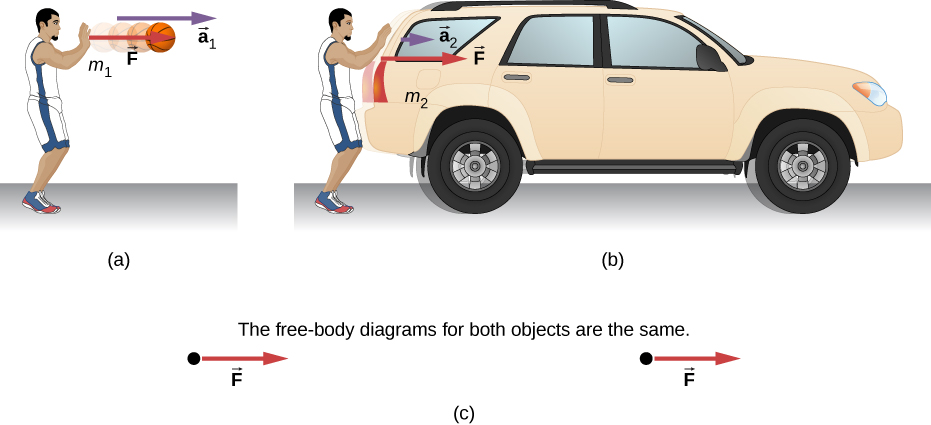It has been found that the acceleration of an object depends only on the net external force and the mass of the object. Combining the two proportionalities just given yields Newton’s second law.

Newton’s Second Law of Motion

The acceleration of a system is directly proportional to and in the same direction as the net external force acting on the system and is inversely proportion to its mass. In equation form, Newton’s second law is

$\stackrel{\to }{a}=\frac{{\stackrel{\to }{F}}_{\text{net}}}{m},$

where $\stackrel{\to }{a}$ is the acceleration, ${\stackrel{\to }{F}}_{\text{net}}$ is the net force, and m is the mass. This is often written in the more familiar form

${\stackrel{\to }{F}}_{\text{net}}=\sum \stackrel{\to }{F}=m\stackrel{\to }{a},$

but the first equation gives more insight into what Newton’s second law means. When only the magnitude of force and acceleration are considered, this equation can be written in the simpler scalar form:

${F}_{\text{net}}=ma.$

The law is a cause-and-effect relationship among three quantities that is not simply based on their definitions. The validity of the second law is based on experimental verification. The free-body diagram, which you will learn to draw in Drawing Free-Body Diagrams, is the basis for writing Newton’s second law.

What Acceleration Can a Person Produce When Pushing a Lawn Mower?
Suppose that the net external force (push minus friction) exerted on a lawn mower is 51 N (about 11 lb.) parallel to the ground ((Figure)). The mass of the mower is 24 kg. What is its acceleration?

(a) The net force on a lawn mower is 51 N to the right. At what rate does the lawn mower accelerate to the right? (b) The free-body diagram for this problem is shown.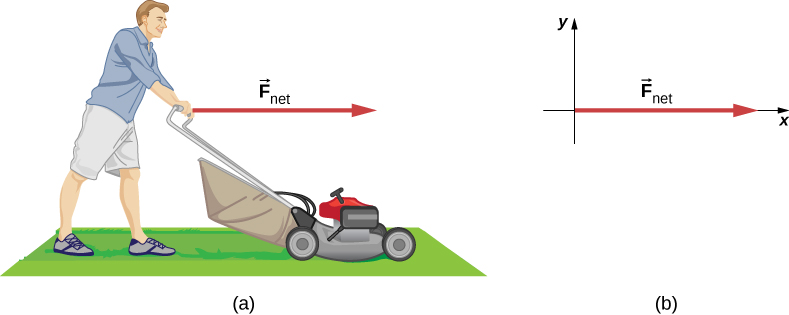Strategy
This problem involves only motion in the horizontal direction; we are also given the net force, indicated by the single vector, but we can suppress the vector nature and concentrate on applying Newton’s second law. Since ${F}_{\text{net}}$ and m are given, the acceleration can be calculated directly from Newton’s second law as ${F}_{\text{net}}=ma.$

Solution
The magnitude of the acceleration a is $a={F}_{\text{net}}\text{/}m$. Entering known values gives

$a=\frac{51\phantom{\rule{0.2em}{0ex}}\text{N}}{24\phantom{\rule{0.2em}{0ex}}\text{kg}}.$

Substituting the unit of kilograms times meters per square second for newtons yields

$a=\frac{51\phantom{\rule{0.2em}{0ex}}\text{kg}·{\text{m/s}}^{2}}{24\phantom{\rule{0.2em}{0ex}}\text{kg}}=2.1\phantom{\rule{0.2em}{0ex}}{\text{m/s}}^{2}.$

Significance
The direction of the acceleration is the same direction as that of the net force, which is parallel to the ground. This is a result of the vector relationship expressed in Newton’s second law, that is, the vector representing net force is the scalar multiple of the acceleration vector. There is no information given in this example about the individual external forces acting on the system, but we can say something about their relative magnitudes. For example, the force exerted by the person pushing the mower must be greater than the friction opposing the motion (since we know the mower moved forward), and the vertical forces must cancel because no acceleration occurs in the vertical direction (the mower is moving only horizontally). The acceleration found is small enough to be reasonable for a person pushing a mower. Such an effort would not last too long, because the person’s top speed would soon be reached.

Check Your Understanding At the time of its launch, the HMS Titanic was the most massive mobile object ever built, with a mass of $6.0\phantom{\rule{0.2em}{0ex}}×\phantom{\rule{0.2em}{0ex}}{10}^{7}\phantom{\rule{0.2em}{0ex}}\text{kg}$. If a force of 6 MN $\left(6\phantom{\rule{0.2em}{0ex}}×\phantom{\rule{0.2em}{0ex}}{10}^{6}\phantom{\rule{0.2em}{0ex}}\text{N}\right)$ was applied to the ship, what acceleration would it experience?

$0.1\phantom{\rule{0.2em}{0ex}}{\text{m/s}}^{2}$

In the preceding example, we dealt with net force only for simplicity. However, several forces act on the lawn mower. The weight $\stackrel{\to }{w}$ (discussed in detail in Mass and Weight) pulls down on the mower, toward the center of Earth; this produces a contact force on the ground. The ground must exert an upward force on the lawn mower, known as the normal force $\stackrel{\to }{N}$, which we define in Common Forces. These forces are balanced and therefore do not produce vertical acceleration. In the next example, we show both of these forces. As you continue to solve problems using Newton’s second law, be sure to show multiple forces.

Which Force Is Bigger?
(a) The car shown in (Figure) is moving at a constant speed. Which force is bigger, ${\stackrel{\to }{F}}_{\text{engine}}$ or ${\stackrel{\to }{F}}_{\text{friction}}$? Explain.

(b) The same car is now accelerating to the right. Which force is bigger, ${\stackrel{\to }{F}}_{\text{engine}}$ or ${\stackrel{\to }{F}}_{\text{friction}}?$ Explain.

A car is shown (a) moving at constant speed and (b) accelerating. How do the forces acting on the car compare in each case? (a) What does the knowledge that the car is moving at constant velocity tell us about the net horizontal force on the car compared to the friction force? (b) What does the knowledge that the car is accelerating tell us about the horizontal force on the car compared to the friction force?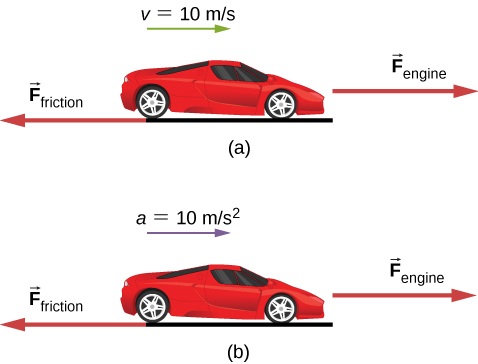Strategy
We must consider Newton’s first and second laws to analyze the situation. We need to decide which law applies; this, in turn, will tell us about the relationship between the forces.

Solution

1. The forces are equal. According to Newton’s first law, if the net force is zero, the velocity is constant.
2. In this case, ${\stackrel{\to }{F}}_{\text{engine}}$ must be larger than ${\stackrel{\to }{F}}_{\text{friction}}.$ According to Newton’s second law, a net force is required to cause acceleration.

Significance
These questions may seem trivial, but they are commonly answered incorrectly. For a car or any other object to move, it must be accelerated from rest to the desired speed; this requires that the engine force be greater than the friction force. Once the car is moving at constant velocity, the net force must be zero; otherwise, the car will accelerate (gain speed). To solve problems involving Newton’s laws, we must understand whether to apply Newton’s first law (where $\sum \stackrel{\to }{F}=\stackrel{\to }{0}$) or Newton’s second law (where $\sum \stackrel{\to }{F}$ is not zero). This will be apparent as you see more examples and attempt to solve problems on your own.

What Rocket Thrust Accelerates This Sled?
Before manned space flights, rocket sleds were used to test aircraft, missile equipment, and physiological effects on human subjects at high speeds. They consisted of a platform that was mounted on one or two rails and propelled by several rockets.

Calculate the magnitude of force exerted by each rocket, called its thrust T, for the four-rocket propulsion system shown in (Figure). The sled’s initial acceleration is $49\phantom{\rule{0.2em}{0ex}}{\text{m/s}}^{2}$, the mass of the system is 2100 kg, and the force of friction opposing the motion is 650 N.

A sled experiences a rocket thrust that accelerates it to the right. Each rocket creates an identical thrust T. The system here is the sled, its rockets, and its rider, so none of the forces between these objects are considered. The arrow representing friction $\left(\stackrel{\to }{f}\right)$ is drawn larger than scale.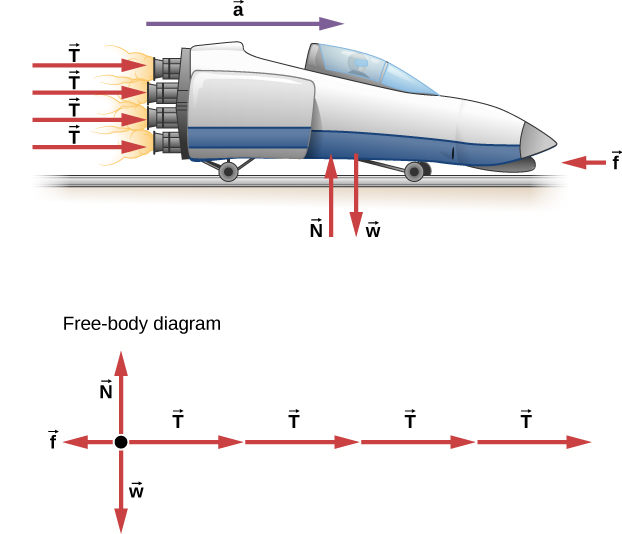Strategy
Although forces are acting both vertically and horizontally, we assume the vertical forces cancel because there is no vertical acceleration. This leaves us with only horizontal forces and a simpler one-dimensional problem. Directions are indicated with plus or minus signs, with right taken as the positive direction. See the free-body diagram in (Figure).

Solution
Since acceleration, mass, and the force of friction are given, we start with Newton’s second law and look for ways to find the thrust of the engines. We have defined the direction of the force and acceleration as acting “to the right,” so we need to consider only the magnitudes of these quantities in the calculations. Hence we begin with

${F}_{\text{net}}=ma$

where ${F}_{\text{net}}$ is the net force along the horizontal direction. We can see from the figure that the engine thrusts add, whereas friction opposes the thrust. In equation form, the net external force is

${F}_{\text{net}}=4T-f.$

Substituting this into Newton’s second law gives us

${F}_{\text{net}}=ma=4T-f.$

Using a little algebra, we solve for the total thrust 4T:

$4T=ma+f.$

Substituting known values yields

$4T=ma+f=\left(2100\phantom{\rule{0.2em}{0ex}}\text{kg}\right)\left(49\phantom{\rule{0.2em}{0ex}}{\text{m}\text{/}\text{s}}^{2}\right)+650\phantom{\rule{0.2em}{0ex}}\text{N}.$

Therefore, the total thrust is

$4T=1.0\phantom{\rule{0.2em}{0ex}}×\phantom{\rule{0.2em}{0ex}}{10}^{5}\phantom{\rule{0.2em}{0ex}}\text{N},$

and the individual thrusts are

$T=\frac{1.0\phantom{\rule{0.2em}{0ex}}×\phantom{\rule{0.2em}{0ex}}{10}^{5}\phantom{\rule{0.2em}{0ex}}\text{N}}{4}=2.5\phantom{\rule{0.2em}{0ex}}×\phantom{\rule{0.2em}{0ex}}{10}^{4}\phantom{\rule{0.2em}{0ex}}\text{N}.$

Significance
The numbers are quite large, so the result might surprise you. Experiments such as this were performed in the early 1960s to test the limits of human endurance, and the setup was designed to protect human subjects in jet fighter emergency ejections. Speeds of 1000 km/h were obtained, with accelerations of 45 g’s. (Recall that g, acceleration due to gravity, is $9.80\phantom{\rule{0.2em}{0ex}}{\text{m/s}}^{2}$. When we say that acceleration is 45 g’s, it is $45\phantom{\rule{0.2em}{0ex}}×\phantom{\rule{0.2em}{0ex}}9.8\phantom{\rule{0.2em}{0ex}}{\text{m/s}}^{2},$ which is approximately $440\phantom{\rule{0.2em}{0ex}}{\text{m/s}}^{2}$.) Although living subjects are not used anymore, land speeds of 10,000 km/h have been obtained with a rocket sled.

In this example, as in the preceding one, the system of interest is obvious. We see in later examples that choosing the system of interest is crucial—and the choice is not always obvious.

Newton’s second law is more than a definition; it is a relationship among acceleration, force, and mass. It can help us make predictions. Each of those physical quantities can be defined independently, so the second law tells us something basic and universal about nature.

Check Your Understanding A 550-kg sports car collides with a 2200-kg truck, and during the collision, the net force on each vehicle is the force exerted by the other. If the magnitude of the truck’s acceleration is $10\phantom{\rule{0.2em}{0ex}}{\text{m/s}}^{2},$ what is the magnitude of the sports car’s acceleration?

$40\phantom{\rule{0.2em}{0ex}}{\text{m/s}}^{2}$

### Component Form of Newton’s Second Law

We have developed Newton’s second law and presented it as a vector equation in (Figure). This vector equation can be written as three component equations:

$\sum {\stackrel{\to }{F}}_{x}=m{\stackrel{\to }{a}}_{x},\phantom{\rule{0.2em}{0ex}}\sum {\stackrel{\to }{F}}_{y}=m{\stackrel{\to }{a}}_{y},\phantom{\rule{0.2em}{0ex}}\text{and}\phantom{\rule{0.2em}{0ex}}\sum {\stackrel{\to }{F}}_{z}=m{\stackrel{\to }{a}}_{z}.$

The second law is a description of how a body responds mechanically to its environment. The influence of the environment is the net force ${\stackrel{\to }{F}}_{\text{net}},$ the body’s response is the acceleration $\stackrel{\to }{a},$ and the strength of the response is inversely proportional to the mass m. The larger the mass of an object, the smaller its response (its acceleration) to the influence of the environment (a given net force). Therefore, a body’s mass is a measure of its inertia, as we explained in Newton’s First Law.

Force on a Soccer Ball
A 0.400-kg soccer ball is kicked across the field by a player; it undergoes acceleration given by $\stackrel{\to }{a}=3.00\stackrel{^}{i}+7.00\stackrel{^}{j}\phantom{\rule{0.2em}{0ex}}{\text{m/s}}^{2}.$ Find (a) the resultant force acting on the ball and (b) the magnitude and direction of the resultant force.

Strategy
The vectors in $\stackrel{^}{i}$ and $\stackrel{^}{j}$ format, which indicate force direction along the x-axis and the y-axis, respectively, are involved, so we apply Newton’s second law in vector form.

Solution

1. We apply Newton’s second law:
${\stackrel{\to }{F}}_{\text{net}}=m\stackrel{\to }{a}=\left(0.400\phantom{\rule{0.2em}{0ex}}\text{kg}\right)\left(3.00\stackrel{^}{i}+7.00\stackrel{^}{j}\phantom{\rule{0.2em}{0ex}}{\text{m/s}}^{2}\right)=1.20\stackrel{^}{i}+2.80\stackrel{^}{j}\phantom{\rule{0.2em}{0ex}}\text{N}.$
2. Magnitude and direction are found using the components of ${\stackrel{\to }{F}}_{\text{net}}$:
${F}_{\text{net}}=\sqrt{{\left(1.20\phantom{\rule{0.2em}{0ex}}\text{N}\right)}^{2}+{\left(2.80\phantom{\rule{0.2em}{0ex}}\text{N}\right)}^{2}}=3.05\phantom{\rule{0.2em}{0ex}}\text{N}\phantom{\rule{0.2em}{0ex}}\text{and}\phantom{\rule{0.2em}{0ex}}\theta ={\text{tan}}^{-1}\left(\frac{2.80}{1.20}\right)=66.8\text{°}.$

Significance
We must remember that Newton’s second law is a vector equation. In (a), we are multiplying a vector by a scalar to determine the net force in vector form. While the vector form gives a compact representation of the force vector, it does not tell us how “big” it is, or where it goes, in intuitive terms. In (b), we are determining the actual size (magnitude) of this force and the direction in which it travels.

Mass of a Car
Find the mass of a car if a net force of $-600.0\stackrel{^}{j}\phantom{\rule{0.2em}{0ex}}\text{N}$ produces an acceleration of $-0.2\stackrel{^}{j}\phantom{\rule{0.2em}{0ex}}{\text{m/s}}^{2}$.

Strategy
Vector division is not defined, so $m={\stackrel{\to }{F}}_{\text{net}}\text{/}\stackrel{\to }{a}$ cannot be performed. However, mass m is a scalar, so we can use the scalar form of Newton’s second law, $m={F}_{\text{net}}\text{/}a$.

Solution
We use $m={F}_{\text{net}}\text{/}a$ and substitute the magnitudes of the two vectors: ${F}_{\text{net}}=600.0\phantom{\rule{0.2em}{0ex}}\text{N}$ and $a=0.2\phantom{\rule{0.2em}{0ex}}{\text{m/s}}^{2}.$ Therefore,

$m=\frac{{F}_{\text{net}}}{a}=\frac{600.0\phantom{\rule{0.2em}{0ex}}\text{N}}{0.2\phantom{\rule{0.2em}{0ex}}{\text{m/s}}^{2}}=3000\phantom{\rule{0.2em}{0ex}}\text{kg}.$

Significance
Force and acceleration were given in the $\stackrel{^}{i}$ and $\stackrel{^}{j}$ format, but the answer, mass m, is a scalar and thus is not given in $\stackrel{^}{i}$ and $\stackrel{^}{j}$ form.

Several Forces on a Particle
A particle of mass $m=4.0\phantom{\rule{0.2em}{0ex}}\text{kg}$ is acted upon by four forces of magnitudes. ${F}_{1}=10.0\phantom{\rule{0.2em}{0ex}}\text{N},\phantom{\rule{0.2em}{0ex}}{F}_{2}=40.0\phantom{\rule{0.2em}{0ex}}\text{N},\phantom{\rule{0.2em}{0ex}}{F}_{3}=5.0\phantom{\rule{0.2em}{0ex}}\text{N},\phantom{\rule{0.2em}{0ex}}\text{and}\phantom{\rule{0.2em}{0ex}}{F}_{4}=2.0\phantom{\rule{0.2em}{0ex}}\text{N}$, with the directions as shown in the free-body diagram in (Figure). What is the acceleration of the particle?

Four forces in the xy-plane are applied to a 4.0-kg particle.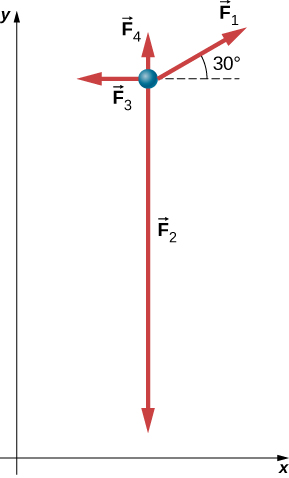Strategy
Because this is a two-dimensional problem, we must use a free-body diagram. First, ${\stackrel{\to }{F}}_{1}$ must be resolved into x– and y-components. We can then apply the second law in each direction.

Solution
We draw a free-body diagram as shown in (Figure). Now we apply Newton’s second law. We consider all vectors resolved into x– and y-components:

$\begin{array}{cccc}\sum {F}_{x}=m{a}_{x}\hfill & & & \sum {F}_{y}=m{a}_{y}\hfill \\ {F}_{1x}-{F}_{3x}=m{a}_{x}\hfill & & & {F}_{1y}+{F}_{4y}-{F}_{2y}=m{a}_{y}\hfill \\ {F}_{1}\phantom{\rule{0.2em}{0ex}}\text{cos}\phantom{\rule{0.2em}{0ex}}30\text{°}-{F}_{3x}=m{a}_{x}\hfill & & & {F}_{1}\text{sin}\phantom{\rule{0.2em}{0ex}}30\text{°}+{F}_{4y}-{F}_{2y}=m{a}_{y}\hfill \\ \left(10.0\phantom{\rule{0.2em}{0ex}}\text{N}\right)\left(\text{cos}\phantom{\rule{0.2em}{0ex}}30\text{°}\right)-5.0\phantom{\rule{0.2em}{0ex}}\text{N}=\left(4.0\phantom{\rule{0.2em}{0ex}}\text{kg}\right){a}_{x}\hfill & & & \left(10.0\phantom{\rule{0.2em}{0ex}}\text{N}\right)\left(\text{sin}\phantom{\rule{0.2em}{0ex}}30\text{°}\right)+2.0\phantom{\rule{0.2em}{0ex}}\text{N}-40.0\phantom{\rule{0.2em}{0ex}}\text{N}=\left(4.0\phantom{\rule{0.2em}{0ex}}\text{kg}\right){a}_{y}\hfill \\ {a}_{x}=0.92\phantom{\rule{0.2em}{0ex}}\text{m}\text{/}{\text{s}}^{2}.\hfill & & & {a}_{y}=-8.3\phantom{\rule{0.2em}{0ex}}{\text{m}\text{/}\text{s}}^{2}.\hfill \end{array}$

Thus, the net acceleration is

$\stackrel{\to }{a}=\left(0.92\stackrel{^}{i}-8.3\stackrel{^}{j}\right)\phantom{\rule{0.2em}{0ex}}\text{m}\text{/}{\text{s}}^{2},$

which is a vector of magnitude $8.4\phantom{\rule{0.2em}{0ex}}{\text{m/s}}^{2}$ directed at $276\text{°}$ to the positive x-axis.

Significance
Numerous examples in everyday life can be found that involve three or more forces acting on a single object, such as cables running from the Golden Gate Bridge or a football player being tackled by three defenders. We can see that the solution of this example is just an extension of what we have already done.

Check Your Understanding A car has forces acting on it, as shown below. The mass of the car is 1000.0 kg. The road is slick, so friction can be ignored. (a) What is the net force on the car? (b) What is the acceleration of the car?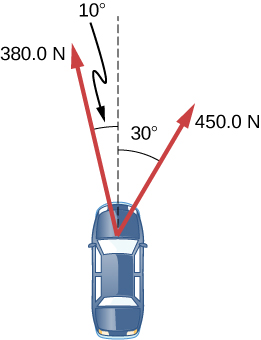a. $159.0\stackrel{^}{i}+770.0\stackrel{^}{j}\phantom{\rule{0.2em}{0ex}}\text{N}$; b. $0.1590\stackrel{^}{i}+0.7700\stackrel{^}{j}\phantom{\rule{0.2em}{0ex}}\text{N}$

### Newton’s Second Law and Momentum

Newton actually stated his second law in terms of momentum: “The instantaneous rate at which a body’s momentum changes is equal to the net force acting on the body.” (“Instantaneous rate” implies that the derivative is involved.) This can be given by the vector equation

${\stackrel{\to }{F}}_{\text{net}}=\frac{d\stackrel{\to }{p}}{dt}.$

This means that Newton’s second law addresses the central question of motion: What causes a change in motion of an object? Momentum was described by Newton as “quantity of motion,” a way of combining both the velocity of an object and its mass. We devote Linear Momentum and Collisions to the study of momentum.

For now, it is sufficient to define momentum $\stackrel{\to }{p}$ as the product of the mass of the object m and its velocity $\stackrel{\to }{v}$:

$\stackrel{\to }{p}=m\stackrel{\to }{v}.$

Since velocity is a vector, so is momentum.

It is easy to visualize momentum. A train moving at 10 m/s has more momentum than one that moves at 2 m/s. In everyday life, we speak of one sports team as “having momentum” when they score points against the opposing team.

If we substitute (Figure) into (Figure), we obtain

${\stackrel{\to }{F}}_{\text{net}}=\frac{d\stackrel{\to }{p}}{dt}=\frac{d\left(m\stackrel{\to }{v}\right)}{dt}.$

When m is constant, we have

${\stackrel{\to }{F}}_{\text{net}}=m\frac{d\left(\stackrel{\to }{v}\right)}{dt}=m\stackrel{\to }{a}.$

Thus, we see that the momentum form of Newton’s second law reduces to the form given earlier in this section.

Explore the forces at work when pulling a cart or pushing a refrigerator, crate, or person. Create an applied force and see how it makes objects move. Put an object on a ramp and see how it affects its motion.

### Summary

• An external force acts on a system from outside the system, as opposed to internal forces, which act between components within the system.
• Newton’s second law of motion says that the net external force on an object with a certain mass is directly proportional to and in the same direction as the acceleration of the object.
• Newton’s second law can also describe net force as the instantaneous rate of change of momentum. Thus, a net external force causes nonzero acceleration.

### Conceptual Questions

Why can we neglect forces such as those holding a body together when we apply Newton’s second law?

A rock is thrown straight up. At the top of the trajectory, the velocity is momentarily zero. Does this imply that the force acting on the object is zero? Explain your answer.

No. If the force were zero at this point, then there would be nothing to change the object’s momentary zero velocity. Since we do not observe the object hanging motionless in the air, the force could not be zero.

### Problems

Andrea, a 63.0-kg sprinter, starts a race with an acceleration of $4.200\phantom{\rule{0.2em}{0ex}}{\text{m/s}}^{2}$. What is the net external force on her?

If the sprinter from the previous problem accelerates at that rate for 20.00 m and then maintains that velocity for the remainder of a 100.00-m dash, what will her time be for the race?

Running from rest, the sprinter attains a velocity of $v=12.96\phantom{\rule{0.2em}{0ex}}\text{m/s}$, at end of acceleration. We find the time for acceleration using $x=20.00\phantom{\rule{0.2em}{0ex}}\text{m}=0+0.5a{t}_{1}{}^{2}$, or ${t}_{1}=3.086\phantom{\rule{0.2em}{0ex}}\text{s.}$ For maintained velocity, ${x}_{2}=v{t}_{2}$, or ${t}_{2}={x}_{2}\text{/}v=80.00\phantom{\rule{0.2em}{0ex}}\text{m}\text{/}12.96\phantom{\rule{0.2em}{0ex}}\text{m}\text{/}\text{s}=6.173\phantom{\rule{0.2em}{0ex}}\text{s}$. $\text{Total time}=9.259\phantom{\rule{0.2em}{0ex}}\text{s}$.

A cleaner pushes a 4.50-kg laundry cart in such a way that the net external force on it is 60.0 N. Calculate the magnitude of his cart’s acceleration.

Astronauts in orbit are apparently weightless. This means that a clever method of measuring the mass of astronauts is needed to monitor their mass gains or losses, and adjust their diet. One way to do this is to exert a known force on an astronaut and measure the acceleration produced. Suppose a net external force of 50.0 N is exerted, and an astronaut’s acceleration is measured to be $0.893\phantom{\rule{0.2em}{0ex}}{\text{m/s}}^{2}$. (a) Calculate her mass. (b) By exerting a force on the astronaut, the vehicle in which she orbits experiences an equal and opposite force. Use this knowledge to find an equation for the acceleration of the system (astronaut and spaceship) that would be measured by a nearby observer. (c) Discuss how this would affect the measurement of the astronaut’s acceleration. Propose a method by which recoil of the vehicle is avoided.

a. $m=56.0\phantom{\rule{0.2em}{0ex}}\text{kg}$; b. ${a}_{\text{meas}}={a}_{\text{astro}}+{a}_{\text{ship}},\phantom{\rule{0.2em}{0ex}}\text{where}\phantom{\rule{0.2em}{0ex}}{a}_{\text{ship}}=\frac{{m}_{\text{astro}}{a}_{\text{astro}}}{{m}_{\text{ship}}}$; c. If the force could be exerted on the astronaut by another source (other than the spaceship), then the spaceship would not experience a recoil.

In (Figure), the net external force on the 24-kg mower is given as 51 N. If the force of friction opposing the motion is 24 N, what force F (in newtons) is the person exerting on the mower? Suppose the mower is moving at 1.5 m/s when the force F is removed. How far will the mower go before stopping?

The rocket sled shown below decelerates at a rate of $196\phantom{\rule{0.2em}{0ex}}{\text{m/s}}^{2}$. What force is necessary to produce this deceleration? Assume that the rockets are off. The mass of the system is $2.10\phantom{\rule{0.2em}{0ex}}×\phantom{\rule{0.2em}{0ex}}{10}^{3}$ kg.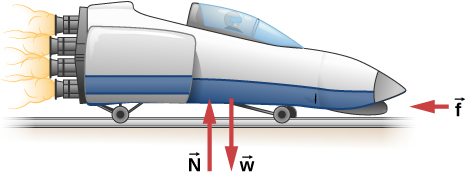${F}_{\text{net}}=4.12\phantom{\rule{0.2em}{0ex}}×\phantom{\rule{0.2em}{0ex}}{10}^{5}\phantom{\rule{0.2em}{0ex}}\text{N}$

If the rocket sled shown in the previous problem starts with only one rocket burning, what is the magnitude of this acceleration? Assume that the mass of the system is $2.10\phantom{\rule{0.2em}{0ex}}×\phantom{\rule{0.2em}{0ex}}{10}^{3}$ kg, the thrust T is $2.40\phantom{\rule{0.2em}{0ex}}×\phantom{\rule{0.2em}{0ex}}{10}^{4}\phantom{\rule{0.2em}{0ex}}\text{N},$ and the force of friction opposing the motion is 650.0 N. (b) Why is the acceleration not one-fourth of what it is with all rockets burning?

What is the deceleration of the rocket sled if it comes to rest in 1.10 s from a speed of 1000.0 km/h? (Such deceleration caused one test subject to black out and have temporary blindness.)

$a={253\phantom{\rule{0.2em}{0ex}}\text{m/s}}^{2}$

Suppose two children push horizontally, but in exactly opposite directions, on a third child in a wagon. The first child exerts a force of 75.0 N, the second exerts a force of 90.0 N, friction is 12.0 N, and the mass of the third child plus wagon is 23.0 kg. (a) What is the system of interest if the acceleration of the child in the wagon is to be calculated? (See the free-body diagram.) (b) Calculate the acceleration. (c) What would the acceleration be if friction were 15.0 N?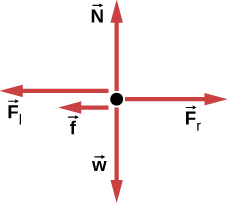A powerful motorcycle can produce an acceleration of $3.50\phantom{\rule{0.2em}{0ex}}{\text{m/s}}^{2}$ while traveling at 90.0 km/h. At that speed, the forces resisting motion, including friction and air resistance, total 400.0 N. (Air resistance is analogous to air friction. It always opposes the motion of an object.) What is the magnitude of the force that motorcycle exerts backward on the ground to produce its acceleration if the mass of the motorcycle with rider is 245 kg?

${F}_{\text{net}}=F-f=ma⇒F=1.26\phantom{\rule{0.2em}{0ex}}×\phantom{\rule{0.2em}{0ex}}{10}^{3}\phantom{\rule{0.2em}{0ex}}\text{N}$

A car with a mass of 1000.0 kg accelerates from 0 to 90.0 km/h in 10.0 s. (a) What is its acceleration? (b) What is the net force on the car?

The driver in the previous problem applies the brakes when the car is moving at 90.0 km/h, and the car comes to rest after traveling 40.0 m. What is the net force on the car during its deceleration?

$\begin{array}{}\\ \\ {v}^{2}={v}_{0}^{2}+2ax⇒a=\text{−}7.80\phantom{\rule{0.2em}{0ex}}{\text{m/s}}^{2}\hfill \\ {F}_{\text{net}}=-7.80\phantom{\rule{0.2em}{0ex}}×\phantom{\rule{0.2em}{0ex}}{10}^{3}\phantom{\rule{0.2em}{0ex}}\text{N}\hfill \end{array}$

An 80.0-kg passenger in an SUV traveling at $1.00\phantom{\rule{0.2em}{0ex}}×\phantom{\rule{0.2em}{0ex}}{10}^{3}$ km/h is wearing a seat belt. The driver slams on the brakes and the SUV stops in 45.0 m. Find the force of the seat belt on the passenger.

A particle of mass 2.0 kg is acted on by a single force ${\stackrel{\to }{F}}_{1}=18\stackrel{^}{i}\phantom{\rule{0.2em}{0ex}}\text{N}.$ (a) What is the particle’s acceleration? (b) If the particle starts at rest, how far does it travel in the first 5.0 s?

a. ${\stackrel{\to }{F}}_{\text{net}}=m\stackrel{\to }{a}⇒\stackrel{\to }{a}=9.0\stackrel{^}{i}\phantom{\rule{0.2em}{0ex}}{\text{m/s}}^{2}$; b. The acceleration has magnitude $9.0\phantom{\rule{0.2em}{0ex}}{\text{m/s}}^{2}$, so $x=110\phantom{\rule{0.2em}{0ex}}\text{m}$.

Suppose that the particle of the previous problem also experiences forces ${\stackrel{\to }{F}}_{2}=-15\stackrel{^}{i}\phantom{\rule{0.2em}{0ex}}\text{N}$ and ${\stackrel{\to }{F}}_{3}=6.0\stackrel{^}{j}\phantom{\rule{0.2em}{0ex}}\text{N}.$ What is its acceleration in this case?

Find the acceleration of the body of mass 5.0 kg shown below.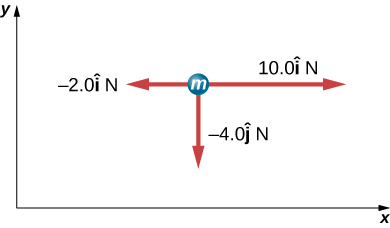$1.6\stackrel{^}{i}-0.8\stackrel{^}{j}\phantom{\rule{0.2em}{0ex}}{\text{m/s}}^{2}$

In the following figure, the horizontal surface on which this block slides is frictionless. If the two forces acting on it each have magnitude $F=30.0\phantom{\rule{0.2em}{0ex}}\text{N}$ and $M=10.0\phantom{\rule{0.2em}{0ex}}\text{kg}$, what is the magnitude of the resulting acceleration of the block?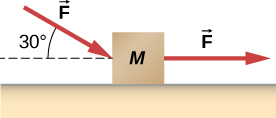### Glossary

Newton’s second law of motion
acceleration of a system is directly proportional to and in the same direction as the net external force acting on the system and is inversely proportional to its mass